# Canonical sections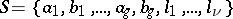ofcurves on a finite Riemann surfaceof genuswith a boundary ofcomponents such that when these curves are removed from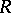, i.e. on cuttingalong the curves of, there remains a (planar) simply-connected domain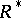. More precisely, a systemis a set of canonical sections if to each closed or cyclic section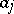,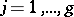, in(or cycle for short) there is exactly one so-called adjoint cycle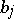cuttingat exactly one fixed point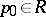common to all the sections of. The remaining cycles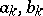,, and curves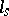,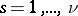, have only the pointin common, and do not pass from one side of the sectionto the other; each curvejoins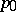with the corresponding boundary component. On a given Riemann surfacethere exists an infinite set of systems of canonical sections. In particular, for any simply-connected domain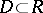that, together with its closure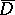, lies strictly in the interior of, a system of canonical sections can be chosen such that.
Furthermore, it is always possible to find a system of canonical sectionsconsisting entirely of analytic curves. The uniqueness of a systemof analytic curves can be ensured, for example, by the additional requirement that some functional related toattains an extremum. In particular, one can draw cyclic canonical sections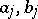of a systemsuch that the greatest value of the Robin constant in the class of systems homotopic tois attained at a pointin a specific domain,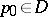. Uniqueness of the curvescan also be ensured by requiring that the Robin constants are maximized at a specified pair of points (see ).